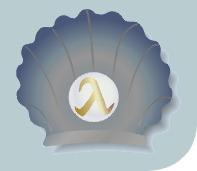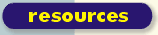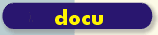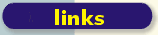scsh.net

# Date Calculation

These snippets contains three small function for calculating the first week-day in a month, whether a year is a leap year, and the number of days in a month.

```  ;; ,open srfi-19

;; return the first weekday in a month as a number
;; (0 for sunday, 1 for monday, ...)

(define (first-week-day month year)
(date-week-day (make-date 0 0 0 0 1 month year 0)))

(define days-per-month-vector
(vector 31 28 31 30 31 30 31 31 30 31 30 31))

(define (leap-year? year)
(cond
((zero? (modulo year 400)) #t)
((zero? (modulo year 100)) #f)
((zero? (modulo year 4)) #t)
(else #f)))

(define (days-in-month month year)
(if (= month 2)
(if (leap-year? year)
29
28)
(vector-ref days-per-month-vector
(- month 1))))

```

-Eric

DateCalculation - raw wiki source | code snippets archive